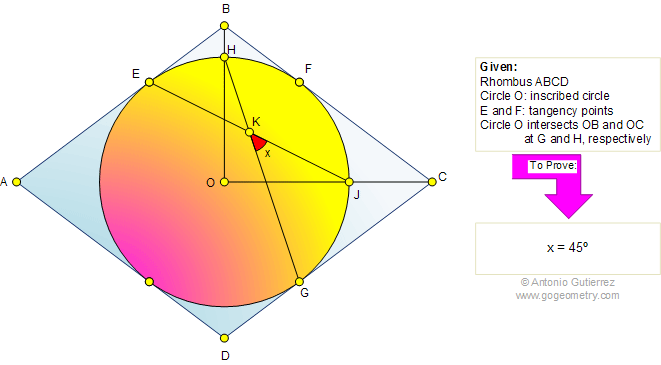Problem 455: Rhombus, Inscribed Circle, Angle, Chord, 45 Degrees The figure shows a rhombus ABCD with the inscribed circle O. Points E, F, and G are the tangency points. Circle O intersects OB and OC at H and J, respectively. If chords EJ and GH meet at K, prove that the measure of angle JKG is 45 degrees.Recent Additions